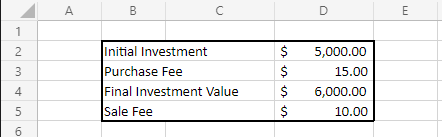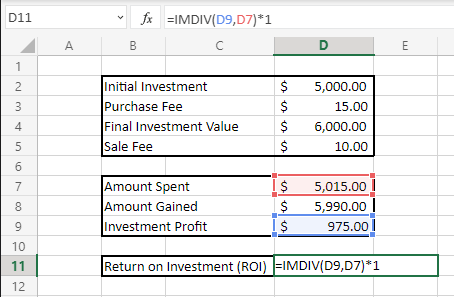# How To Calculate Return On Investment (ROI)

Return on investment (ROI) is a key performance marker for investors to gauge the profitability of their investments. Considering the cost of an investment compared to its return, ROI allows investors like individuals or businesses to make informed financial decisions and maximize the effectiveness of investments.

Expressed as a percentage, ROI can be used to compare various investment opportunities to select the most effective or profitable for an investor. ROI can also be used to calculate the return of previous investments to measure their historical efficiency.

This article will explain the ROI definition and what is a good ROI. This article will also illustrate the ROI equation and demonstrate how to calculate ROI step by step.

## ROI Definition

Return on investment (ROI) is a popular performance metric utilized to measure the effectiveness and profitability of an investment’s performance. As expressed in this ROI definition, this metric considers an investment’s net profit or loss and its initial cost or outlay. Articulated in percentage terms, ROI directly measures the return of a particular investment relative to its cost.

## What Is ROI

### ROI Equation

Return on investment (ROI) is calculated by dividing the return of an investment by the initial cost or outlay of the investment. The return of an investment is found by subtracting the amount spent on an investment from the final amount gained on the investment. The sum of these values then divided by the amount spent and multiplied by 100 to express ROI in percentage terms.

Return on Investment (ROI) = [Amount Gained – Amount SpentAmount Spent] x 100

Where:

Amount Gained = total final value of the investment

Amount Spent = total initial cost of the investment

## How To Calculate ROI

### Calculate ROI

1. Determine the total initial cost and the total final value of the investment.
2. Subtract the total initial cost from the total final value of the investment.
3. Divide the product of Step 2 by the total initial cost.
4. Multiply the product of Step 3 by 100 to express ROI in percentage terms.

### Example

An investor is determining the ROI from a prior investment into a publicly-traded company’s stock. The investor recently sold their shares in the company and liquidated their total investment.

Based on information obtained from the sale of the shares, the investor has gathered the following information:

Initial Investment: \$20,000

Fees from Purchase: \$55

Final Value of Investment: \$26,000

Fees from Sale: \$85

Given this information, the investor can determine the amount spent:

Amount Spent = Total Initial Cost of the Investment

Amount Spent = Initial Investment + Any Additional Fees or Costs

Amount Spent = \$20,000 + \$55

Amount Spent = \$20,055

The investor can also determine the amount gained based on this information:

Amount Gained = Total Final Value of the Investment

Amount Gained = Value of Investment – Any Fees or Costs of Liquidating Investment

Amount Gained = \$26,000 – \$85

Amount Gained = \$25,915

With this information, the investor can populate the ROI equation and begin solving:

ROI = [Amount Gained – Amount SpentAmount Spent] x 100

ROI = [25,915 -20,05520,055] x 100

ROI = [25,915 -20,05520,055] x 100

ROI = [5,86020,055] x 100

ROI = (0.292) x 100

ROI = 29.2%

Here we can see that this investor’s purchase of company stock produced a ROI of 29.2%. This value represents the efficiency and profitability of the investment, as the initial outlay grew by \$5,860 to a final value of \$25,915.

Understanding how to calculate ROI like this is important for investors making forward-facing investment decisions as well. When historical data is present, average ROI can be estimated to compare the profitability of future investments between different opportunities. Using this information allows investors to make the most beneficial investment decision.

## Tips for Calculating ROI

### Tip Number 1:

The ROI of an investment will not always be a positive value. When the amount spent exceeds the amount gained from an investment, a net loss has occurred. This net loss will produce a negative ROI, which investors should actively avoid. Most opportunities with positive ROIs are considered worthwhile, but opportunities with the highest ROIs should be prioritized first.

### Tip Number 2:

The ROI equation does not take the need for liquidity of an investment into consideration. Since the passage of time or the length of an investment holding period are not accounted for in the ROI equation, the equation fails to measure the opportunity costs of investments which are missed when the investment cannot be liquidated. If an investment is selected when evaluating ROI, but does not allow the investor to sell their position over a period of time, any investment opportunities which arise during that time period are lost.

### Tip Number 3:

ROI should not be confused with rate of return (ROR), which accounts for an investment’s time frame. Unlike ROR, ROI does not consider the amount of time during which an investment grows, as there are no values in the ROI equation representing the time period of an investment. Instead, ROI can be used in conjunction with ROR or net present value (NPV) to determine the real rate of return of an investment.

## Calculating ROI Using Microsoft Excel

Microsoft Excel can be used to effectively calculate ROI. For investors making quick decisions between several investments, having a ROI calculator available will decrease the amount of time it takes to determine an investments ROI. When setting up an ROI calculator using Excel, follow these simple steps.

For this example, we will calculate the ROI for a \$5,000 investment, which was later liquidated at a final value of \$6,000, as shown below:Based on this information, the following formulas will be inserted into cells D7 and D8 to determine the amount spent and amount gained from the investment, as follows:

Amount Spent: =SUM(D2:D3)

Amount Gained: =SUM(D4,-D5)After calculating the amount spent and amount gained, the total profit from the investment (the amount gained minus the amount spent) will be determined by inserting the following formula into the corresponding cell D9:

=SUM(D8,-D7)

Once the investment profit has been calculated, the profit will be divided by the amount spent on the investment to determine the overall ROI. The following formula will be inserted into cell D11, as shown below:

=IMDIV(D9,D7)*1## What Is A Good ROI?

For investors wondering “What is a good ROI?”, know that any positive ROI is a good ROI. Any profitable investment is worthwhile, although investments should be measured such that those which will yield higher ROIs are prioritized.

Average profitable ROI varies depending on the industry the investment was made into. For the S&P 500 index, an average ROI is considered to be at least 7%. A rate equal to or higher than this amount is considered a good ROI for investments in the stock market.

Other investments grow at lower rates, such as government bonds or United States treasury bills. Government bonds have an average ROI of over 5-6%, while treasury bills have an average ROI of over 3%. While these rates are lower than that of the S&P 500 index, a ROI around these averages is still considered good for these types of investments.

## ROI Takeaways

Return on investment (ROI) is a profitability gauge used to determine the efficiency of an investment, measuring an investment’s return based on the initial cost or outlay of the investment. As explained in the ROI definition, ROI is written as a percentage and can be used to compare different investment opportunities to select the most profitable option for an investor.When calculating ROI, be sure to consider any additional costs present in the initial outlay or deducted from the final value of an investment, such as brokerage or holding fees. Knowing how to calculate ROI is important when comparing various investment opportunities.

### Surface-enhanced Raman spectroscopy

Surface-enhanced Raman spectroscopy gives the same information as normal Raman spectroscopy but with a significantly enhanced signal. It is also referred to as surface-enhanced Raman

### Mössbauer Spectroscopy

In 1958, a German physicist, Rudolf Mossbauer, invented Mossbauer spectroscopy. It is a versatile technique that provides sensitive information about the structural, magnetic, chemical and

### Energy dispersive spectroscopy (EDS)

Energy dispersive spectroscopy (EDS) is an interesting analytical spectroscopic technique. It is mainly used to characterize and find the relative abundance of different chemical elements